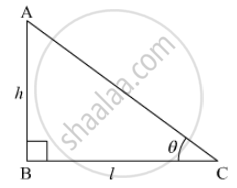Share

# The Ratio of the Height of a Tower and the Length of Its Shadow on the Ground is Sqrt3 : 1. What is the Angle of Elevation of the Sun? - CBSE Class 10 - Mathematics

#### Question

The ratio of the height of a tower and the length of its shadow on the ground is sqrt3 : 1. What is the angle of elevation of the sun?

#### SolutionLet the height of the tower be h m and the length of the shadow on the ground be l m.
The angle of elevation of the sun from the ground be θ

We have h:1  = sqrt3:1

Now In triangleABC

=h/l

= sqrt3/1

= tan 60^@

=> theta = 60^@

Hence, the angle of elevation of the sun will be 60

Is there an error in this question or solution?

#### Video TutorialsVIEW ALL 

Solution The Ratio of the Height of a Tower and the Length of Its Shadow on the Ground is Sqrt3 : 1. What is the Angle of Elevation of the Sun? Concept: Heights and Distances.
S